This codelab will show how to build an text-image search engine with Towhee and Milvus, which means search for matched or related images with the input text.

The basic idea behind our text-image search is the extract embeddings from images and texts using a deep neural network and compare the embeddings with those stored in Milvus. Then to use Towhee, a machine learning framework that allows for creating data processing pipelines, and it also provides predefined operators which implement insert and query operation in Milvus.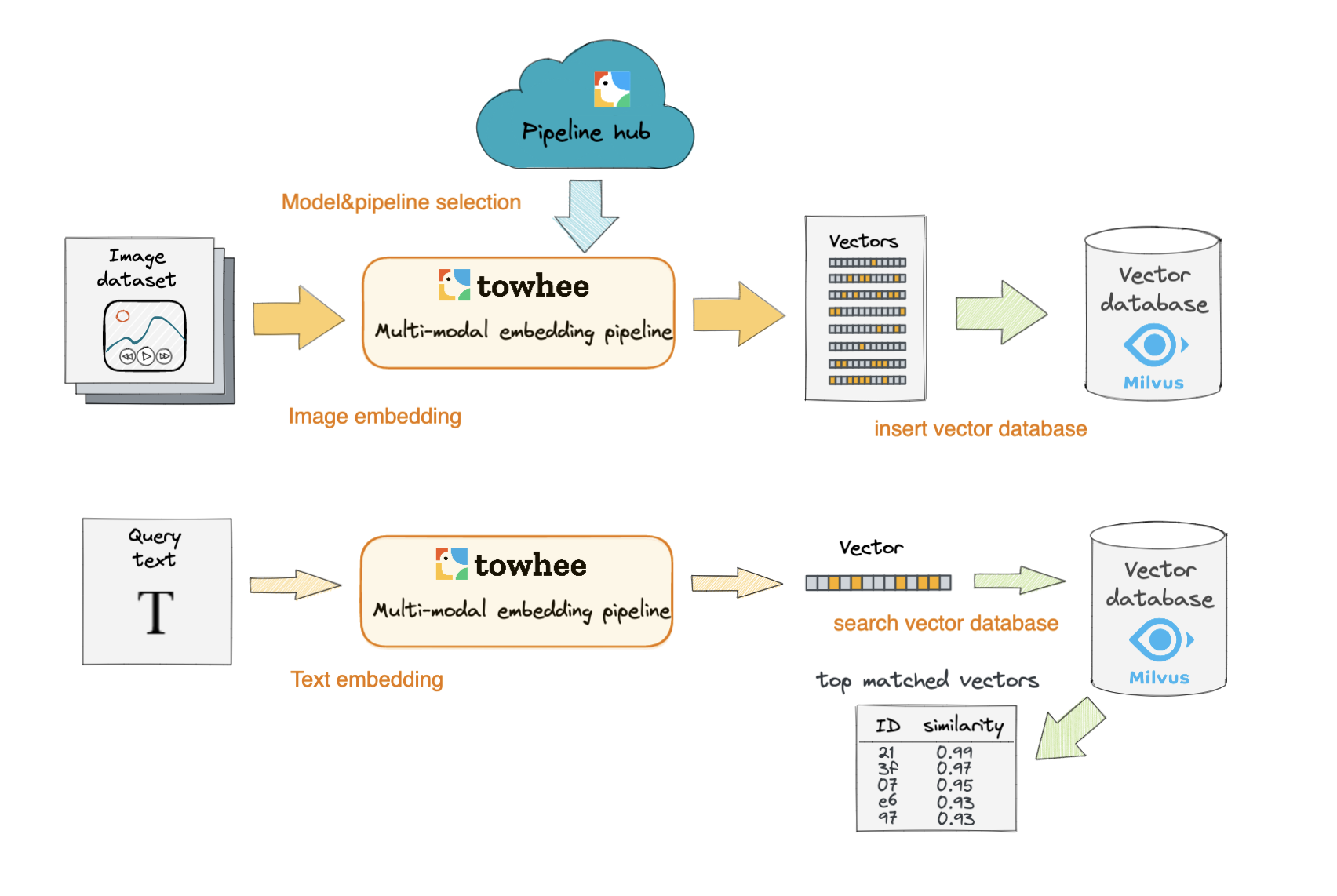## Install Dependencies

First we need to install dependencies such as pymilvus, towhee and gradio.

``````\$ python -m pip install -q pymilvus towhee gradio
``````

## Prepare the data

The dataset used in this demo is a subset of the ImageNet dataset (100 classes, 10 images for each class), and the dataset is available via Github.

The dataset is organized as follows:

• train: directory of candidate images;
• test: directory of test images;
• reverse_image_search.csv: a csv file containing an *id*, *path*, and *label* for each image;

``````\$ curl -L https://github.com/towhee-io/examples/releases/download/data/reverse_image_search.zip -O
\$ unzip -q -o reverse_image_search.zip
``````

Let's take a quick look:

``````import pandas as pd

``````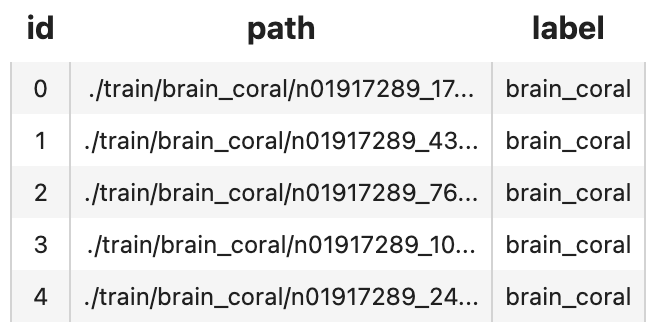To use the dataset for text-image search, let's first define some helper function:

``````import cv2
from towhee._types.image import Image

id_img = df.set_index('id')['path'].to_dict()
imgs = []
for re in results:
path = id_img[re.id]
return imgs
``````

## Create a Milvus Collection

Before getting started, please make sure you have installed milvus. Let's first create a `text_image_search` collection that uses the L2 distance metric and an IVF_FLAT index.

``````from pymilvus import connections, FieldSchema, CollectionSchema, DataType, Collection, utility

connections.connect(host='127.0.0.1', port='19530')

def create_milvus_collection(collection_name, dim):
if utility.has_collection(collection_name):
utility.drop_collection(collection_name)

fields = [
FieldSchema(name='id', dtype=DataType.INT64, descrition='ids', is_primary=True, auto_id=False),
FieldSchema(name='embedding', dtype=DataType.FLOAT_VECTOR, descrition='embedding vectors', dim=dim)
]
schema = CollectionSchema(fields=fields, description='text image search')
collection = Collection(name=collection_name, schema=schema)

# create IVF_FLAT index for collection.
index_params = {
'metric_type':'L2',
'index_type':"IVF_FLAT",
'params':{"nlist":512}
}
collection.create_index(field_name="embedding", index_params=index_params)
return collection

collection = create_milvus_collection('text_image_search', 512)
``````

CLIP Operator can be used generate embeddings for text and image by jointly training an image encoder and text encoder to maximize the cosine similarity.

``````import towhee

towhee.glob['path']('./teddy.png') \
.image_decode['path', 'img']() \
.image_text_embedding.clip['img', 'vec'](model_name='clip_vit_b32', modality='image') \
.tensor_normalize['vec','vec']() \
.select['img', 'vec']() \
.show()
``````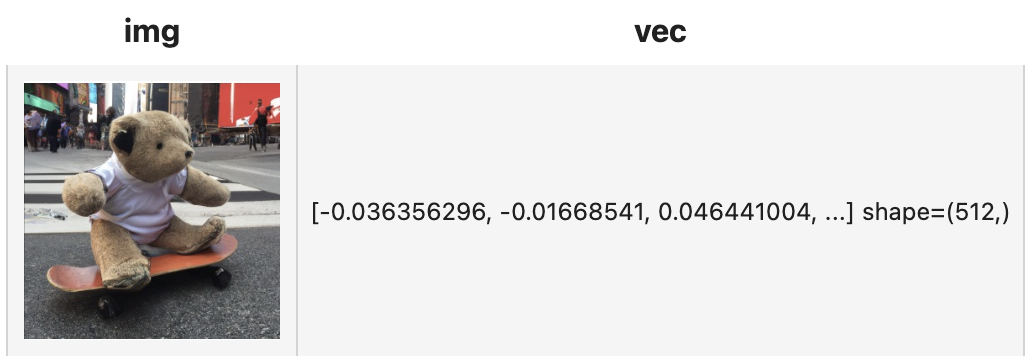And we can set the parameter `modality='text'` to get the text embedding:

``````towhee.dc['text'](["A teddybear on a skateboard in Times Square."]) \
.image_text_embedding.clip['text','vec'](model_name='clip_vit_b32', modality='text') \
.tensor_normalize['vec','vec']() \
.select['text', 'vec']() \
.show()
``````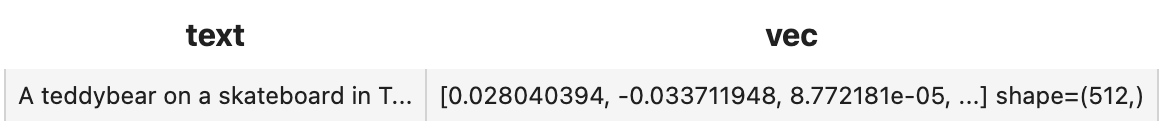Here is detailed explanation of the code:

• `.image_decode['path', 'img']()`: for each row from the data, read and decode the image at `path` and put the pixel data into column `img`;
• `.image_text_embedding.clip['img', 'vec'](model_name='clip_vit_b32',modality='image'/'text')`: extract image or text embedding feature with `image_text_embedding.clip`, an operator from the Towhee hub . This operator supports seveal models including `clip_resnet_r50`,`clip_resnet_r101`,`clip_vit_b32`,`clip_vit_b16`,etc.

We first extract embeddings from images with `clip_vit_32` model and insert the embeddings into Milvus for indexing. Towhee provides a method-chaining style API so that users can assemble a data processing pipeline with operators.

Here is detailed explanation for other apis of the code:

• `towhee.read_csv('reverse_image_search.csv')`: read tabular data from csv file (`id`, `path` and `label` columns);
• `.runas_op['id', 'id'](func=lambda x: int(x))`: for each row from the data, convert the data type of the column `id` from `str` to `int`;
• `.to_milvus['id', 'vec'](collection=collection, batch=100)`: insert image embedding features in to Milvus;
``````collection = create_milvus_collection('text_image_search', 512)

dc = (
.runas_op['id', 'id'](func=lambda x: int(x))
.set_parallel(4)
.image_decode['path', 'img']()
.image_text_embedding.clip['img', 'vec'](model_name='clip_vit_b32', modality='image')
.tensor_normalize['vec','vec']()
.to_milvus['id', 'vec'](collection=collection, batch=100)
)
print('Total number of inserted data is {}.'.format(collection.num_entities))
``````

Total number of inserted data is 1000.

Now that embeddings for candidate images have been inserted into Milvus, we can query across it for nearest neighbors. Again, we use Towhee to load the input Text, compute an embedding vector, and use the vector as a query for Milvus. Because Milvus only outputs image IDs and distance values, we provide a `read_images` function to get the original image based on IDs and display.

``````(
towhee.dc['text'](["A white dog","A black dog"])
.image_text_embedding.clip['text', 'vec'](model_name='clip_vit_b32', modality='text')
.tensor_normalize['vec','vec']()
.milvus_search['vec', 'result'](collection=collection, limit=5)
.select['text', 'result_img']()
.show()
)
``````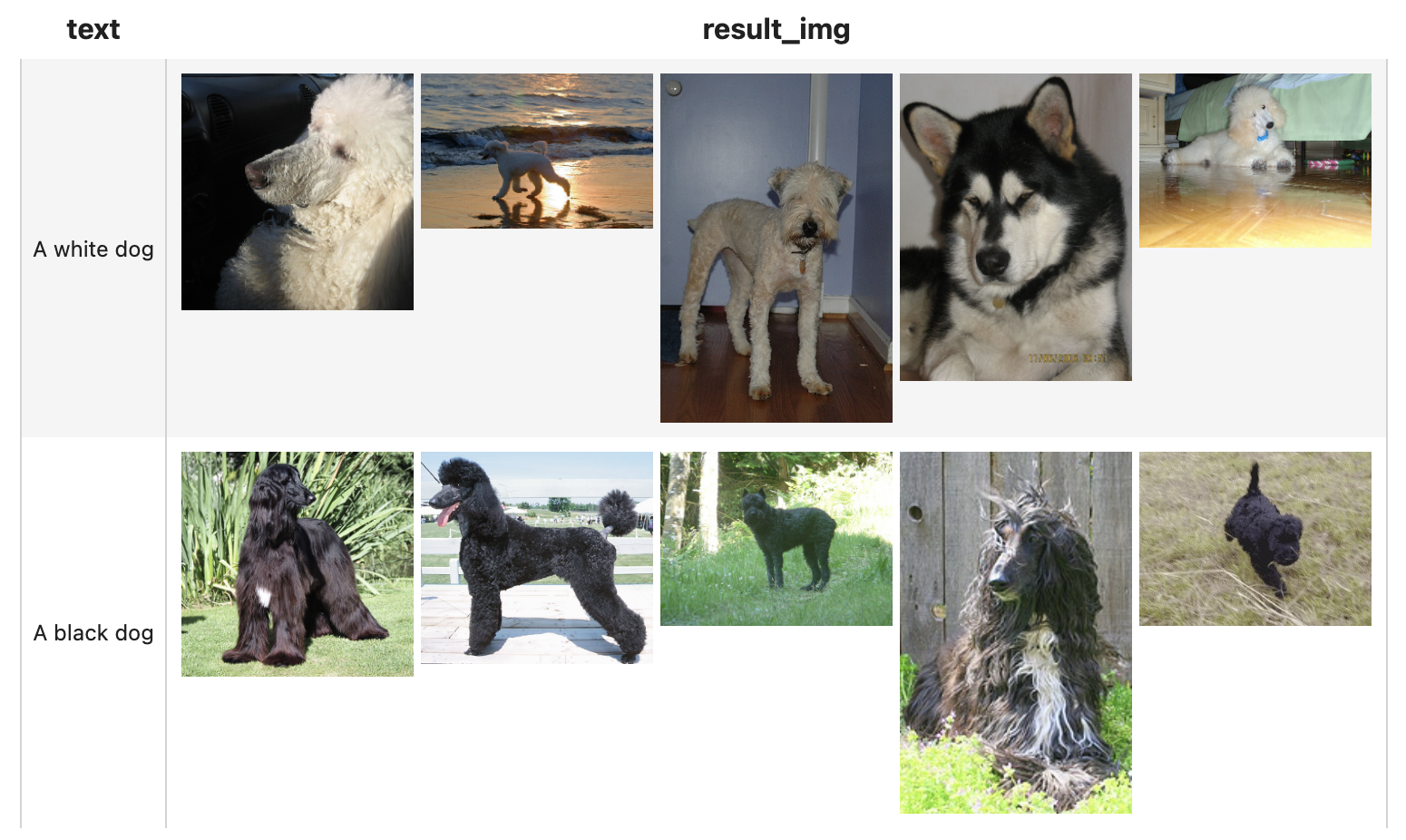We've done an excellent job on the core functionality of our text-image search engine. Now it's time to build a showcase with interface. Gradio is a great tool for building demos. With Gradio, we simply need to wrap the data processing pipeline via a `search_in_milvus` function:

``````with towhee.api() as api:
milvus_search_function = (
api.image_text_embedding.clip(model_name='clip_vit_b32',modality='text')
.tensor_normalize()
.milvus_search(collection='text_image_search', limit=5)
.runas_op(func=lambda res: [id_img[x.id] for x in res])
.as_function()
)